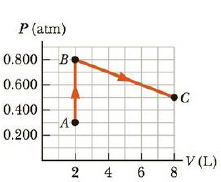Chapter 12, Problem 22P

Chapter
Section
Textbook Problem

A system consisting of 0.025 6 moles of a diatomic ideal gas is taken from state A to state C along the path in Figure P12.22. (a) How much work is done on the gas during this process? (b) What is the lowest temperature of the gas during this process, and where does it occur? (c) Find the change in internal energy of the gas and (d) the energy delivered to the gas in going from A to C. Hint: For part (c), adapt the equation in the remarks of Example 12.9 to a diatomic ideal gas.Figure P12.22

(a)

To determine
The work done on the gas during the process from A to C.

Explanation

Given Info:

The width of the reactangle1 is 6L

The height of the rectangle is 0.500atm

The base of the triangle is 6L

The height of the triangle is 0.300atm

The PV diagram of the ideal gas is,

The area under the path on a PV diagram is equal to the magnitude of the work done on the gas.

Formula to calculate the area under PV diagram is,

A=(W1×H1)+12(B1×H2)

• W1 is the width of the rectangle1
• H1 is the height of the rectangle1
• B1 is the base of triangle2
• H2 is the height of triangle2

Substitute 6L for W1 , 0.500atm for H1 , 6L for B1 and 0

(b)

To determine
what is the lowest temperature of the gas and where does it occur.

(c)

To determine
The change in internal energy of the gas.

(d)

To determine
the energy delivered to the gas.

Still sussing out bartleby?

Check out a sample textbook solution.

See a sample solution

The Solution to Your Study Problems

Bartleby provides explanations to thousands of textbook problems written by our experts, many with advanced degrees!

Get Started

A deficiency of niacin may result in which disease? pellagra beriberi scurvy rickets

Nutrition: Concepts and Controversies - Standalone book (MindTap Course List)

What is meant by the precision of a measurement? How is it indicated?

General Chemistry - Standalone book (MindTap Course List)

What is needed to designate a position?

An Introduction to Physical Science

How are fats and oils structurally similar? How are they different?

Chemistry for Today: General, Organic, and Biochemistry